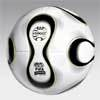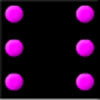#### You may also like### Football World Cup Simulation

A maths-based Football World Cup simulation for teachers and students to use.### Probably ...

You'll need to work in a group for this problem. The idea is to decide, as a group, whether you agree or disagree with each statement.### In the Playground

What can you say about the child who will be first on the playground tomorrow morning at breaktime in your school?

# Winning the Lottery

##### Age 7 to 11 Challenge Level:

We have received many good responses for this question. It is interesting to see all the different lottery rules you have come up with.

Rosalind from Ricards Lodge High School made a good start on the first question:

There are four balls and you must choose one, so it's 1 ball which you choose out of the total of 4 balls that can be chosen. So the chance is 1:4.

Kennard Brentfield Primary School also correctly answered this part of the question.

Another student from Ricards Lodge gave a nice complete solution to the questions:

My method of finding out what the probability is of winning the new lottery, the old lottery game and if it is actually harder to win than the old one is this:

The old lottery game:
In the old lottery game the probability of winning is $\frac{1}{4}$. This is because there are four balls and you pick out one. Therefore you have a chance of $1$ in $4$.

The new lottery game:
The probability of winning the new lottery game is $\frac{1}{6}$. This is because there are six options you could have:
$1$ and $2$, $1$ and $3$, $1$ and $4$, $2$ and $3$, $2$ and $4$, $3$ and $4$.
You can only pick one pair out of the six options so the probability is $1$ in $6$. Therefore it is harder to win the new lottery game than the old one.

If I were going to make a newer even harder lottery game, this would be my method:
There would be four balls and you can pick out any two. Then if your balls were picked out in the same order as you picked out yours then you win!
This is harder because the balls have to be picked out in the correct order making it a lot more difficult to win!

Daisy, also from Ricards Lodge submitted an excellent solution with similar methods. She also investigated the chances of winning if three balls are chosen from four in the bag:

The chance of you winning the lottery in Far away land is $\frac{1}{4}$ because there are only four balls to pick from.
When they made it slightly harder the chance of winning was $\frac{1}{6}$. The possible options you could choose were (without repeating yourself):
$1$ and $2$, $1$ and $3$, $1$ and $4$, $2$ and $3$, $2$ and $4$, $3$ and $4$.
There were only six possible outcomes, therefore your chance of winning was $\frac{1}{6}$.

Using four balls the probability of you winning does not get any harder then $\frac{1}{6}$ unless you need a pattern.
For example if you needed to match with three balls then there are fewer outcomes, in fact only four which brings you back to the probability of $\frac{1}{4}$.

Alistair from Charters School, Krystof from Uhelny Trh, Prague also  sent us good solutions to the first parts of the question.

Katy from Ricards Lodge told us her idea to make the lottery harder, by creating more possible outcomes:

My idea is to have $150$ national lottery balls, only one of them picked. There are $150$ possible outcomes, which is greater than $6$ or $4$ possible outcomes.

Ali from Riversdale Primary also shared how he arrived at his method to make winning more difficult:

I tried to make it harder by picking three balls, but the probability of winning was $1$ in $4$ again, because there is only one ball left in the bag.

To make it harder you have to increase the number of balls. I added one more and found that the chance of winning is $1$ in $5$ if you have to pick one or four balls, or $1$ in $10$ if you have to pick two or three balls.

Alice, Oliver, Michael, Elsa and Rosie from the Extension Maths group at St Nicolas C of E Junior School, Newbury also answered the first two parts of the problem correctly. Alice and Oliver created their own, harder version of the lottery:

Our lottery uses five balls, numbered $1$ to $5$, placed in a bag and you must choose two numbers. Your numbers must match, in the same order, the balls drawn from the bag.
We listed all the possibilities:
$1$ and $2$, $1$ and $3$, $1$ and $4$, $1$ and $5$, $2$ and $1$, $2$ and $3$, $2$ and $4$, $2$ and $5$, $3$ and $1$, $3$ and $2$, $3$ and $4$, $3$ and $5$, $4$ and $1$, $4$ and $2$, $4$ and $3$, $4$ and $5$, $5$ and $1$, $5$ and $2$, $5$ and $3$, $5$ and $4$.
That makes $20$ and they are all equally likely. We know our lottery is harder to win because there are more possibilities. The chances of winning are $\frac{1}{20}$ not $\frac{1}{4}$.

Elsa and Rosie chose a simpler version:
Number of balls: nine Pick: one
Answers: $1$, $2$, $3$, $4$, $5$, $6$, $7$, $8$, $9$
Draw: one ball
Chance of winning: $\frac{1}{9}$
There is one ball picked out of the bag and there are nine balls that have an equally likely chance of being picked. So $9$ will be the denominator. There is one draw so $1$ will be the numerator, so that will equal $\frac{1}{9}$ which means it is harder.

Benjamin from Flora Stevenson Primary School also worked out the problem using the same thinking.

Well done to all of you who sent in your solutions.